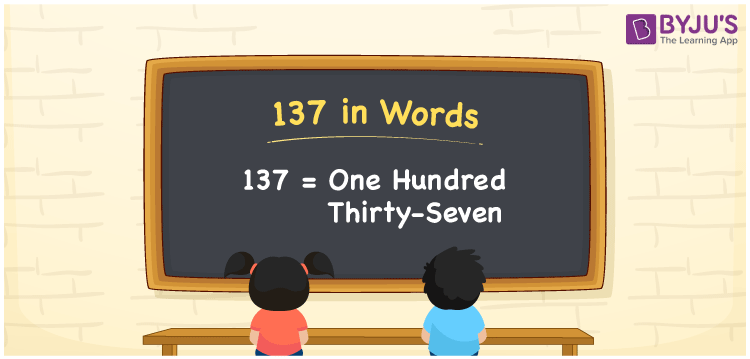# 137 in Words

The number 137 in words is represented by One Hundred Thirty-Seven. For example, Rita saved Rs. 137 while shopping in the market, then you can say, “Rita saved Rupees One Hundred Thirty-Seven while shopping in the market”. 137 is a cardinal number since it represents an exact value or quantity. We will learn how to write the number 137 in words using the English alphabet in this article.

 137 in Words One Hundred Thirty-Seven One Hundred Thirty-Seven in numerical form 137

## 137 in English Words

Generally, we write numbers in words using the letters of the English alphabet in Maths. Therefore, in English, the number 137 is written as One Hundred Thirty-Seven.## How to Write 137 in Words?

137 is a three-digit number. So, let us make a place value chart of up to 3 columns. The place value chart for 137 is given below.

 Hundreds Tens Ones 1 3 7

Hence, we can write the expanded form as:

1 x Hundred + 3 x Ten + 7 x One

= 1 x 100 + 3 x 10 + 7 x 1

= 100 + 30 + 7

= 137

= One Hundred Thirty-Seven

Therefore, 137 in words is written as One Hundred Thirty-Seven

Interesting way of writing 137 in words

1 = One

13 = Thirteen

137 = One Hundred Thirty-Seven

Thus, the word form of the number 137 is One Hundred Thirty-Seven

137 is a natural number that is one less than 138 and one greater than 136

• 137 in words – One Hundred Thirty-Seven
• Is 137 an odd number? – Yes
• Is 137 an even number? – No
• Is 137 a perfect square number? – No
• Is 137 a perfect cube number? – No
• Is 137 a prime number? – Yes
• Is 137 a composite number? – No

## Frequently Asked Questions on 137 in Words

Q1

### How to write 137 in words?

137 in words is written as One Hundred Thirty-Seven.
Q2

### Simplify 100 + 37, and express in words.

Simplifying 100 + 37, we get 137. Therefore, the number 137 in words is One Hundred Thirty-Seven.
Q3

### Write One Hundred Thirty-Seven in numbers.

One Hundred Thirty-Seven in numbers is 137.